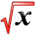# Improper IntegralsThis article is part of the MathHelp Tutoring Wiki

## Improper Integrals Definition

let $f$be integrable on any interval $[a,x]$If $\lim _{x\to +\infty }f(x)$exists and it is a finite number, then $\lim _{x\to +\infty }\int _{a}^{x}f(t)dt=\int _{a}^{\infty }f(t)dt$, and $\int _{a}^{\infty }f(t)dt$is said to be convergent.

### Example1:

Does $\int _{1}^{\infty }{\frac {x}{1+x2}}dx$exist?

Solution: $\lim _{x\to +\infty }\int _{1}^{x}{\frac {t}{1+t^{2}}}dx=\lim _{x\to +\infty }{\frac {1}{2}}\ln {(1+t^{2})}^{x}=\lim _{x\to +\infty }{\frac {1}{2}}(\ln {(1+x^{2})}-\ln {2})=1/2\lim _{x\to \infty }{\frac {1}{2}}\ln {(1+x2)}=\infty$so the integral is divergent

### Example 2:

Check convergence of integration from 2008 to infinity 1/2dt

lim integration from 2008 to x 1/t2dt=lim (-1/x+1/2008)=1/2008

x->infinity x->infinity

so it's convergent

Recall if f,g integrable on [a,b] and f<or=g, integration from a to b f(x)dx<=integration from a to b g(x)dx

## Lamprison Theorem:

If f(x)>=g(x)>=0 for any x

and f,g integrable on any interval, then

(1) If integration from a to infinity f(x)dx convergent => integration from a to infinity g(x)dx is convergent

(2) If integration from a to infinity g(x)dx is divergent => integration from a to infinity f(x)dx is divergent

Q: show that integration from 1 to infinity e^-(x2)dx is convergent for x>1

Solution:

since e^(-x2)<=e-x for any x

because integration from 0 to infinity e-xdx=lim (e-x)/(x-1)=0

x->infinity

so integration from 0 to infinity e-xdx is convergent

then integration from 1 to infinity e-x is convergent

### 2nd kind

Assume interval is finite but f has some discontinuity in the interval, Determine whether integration from a to b f(x)dx is convergent or not?

If limit integration from a to (b-segma) f(x)*dx exists and is finite

segma->o, and segma>0

I= integration from 0 to 1 1/(x-1)*dx

x=1 => discontinuity for f(x)=1/(x-1)

I segma= integration from 0 to (1-segma) 1/(x-1)*dx,

segma>0

I segma=ln|x-1| from 0 to 1-segma=ln|1-segma-1|-ln|0-1|

=ln segma

as segma->0, lim I segma=lim (ln segma)=-infinity

segma-->0 segma-->0

### Conclusion:

Integration from 0 to 1, 1/(x-1)dx is divergent

integration from a to b f(x) is convergent if lim integration from (a+segma) to b f(x)*dx is a finite number

What about integration from a to b f(x)dx if f is discontinuous in C. (a<c<b) either lim f(x) is not finite, or lim f(x) is not finite or both.

$x->c,xc,x>c$In this case, write integration from a to b f(x)dx=integration from a to c f(x)dx+from c to b f(x)dx

## Another example:

Question: For what values of p, the integral of integration from 1 to infinity 1/xp dx is convergent

Translate convergent:

lim(∫ 1 to A)1/x^p*dx= lim x^(1-p)/(1-p) from 1 to A

A->infinity A->infinity

= lim [A^(1-p)/(1-p)-1/(1-p)}

A->infinity

=1/1-p+1/1-p lim A^(1-p)

A->infinity

If 1-p>0, then the limit is infinite

if 1-p<0, then the limit is 0